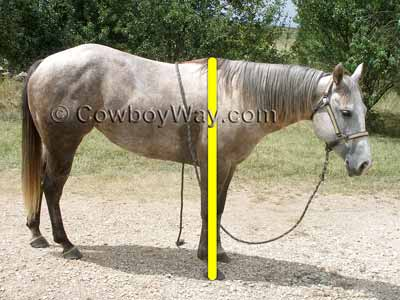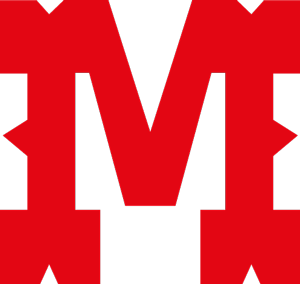# How To Measure Horse Height

It's easy to measure you horse's height accurately.

To determine how tall a horse is measure the horse, in inches, from the ground in a straight line up to the highest point of the withers. This is illustrated in the photo below by the yellow line.

Below: The height of a horse is properly measured, in inches, from the ground to the highest point of the withers.For the most accurate measurement the horse should be on firm, level ground with its front feet even, or close to even.

## Calculating The Results

After you have measured your horse you will need to convert the results from inches to "hands." Horse height is correctly referred to by a unit of measurement known as a "hand." One hand is equal to four inches.

The gray mare in the photo above is 58 inches from the ground to the top of her withers. When 58 is divided by 4, you have 14.5.

The 14 is the number of hands, and the .5 means another half of a hand, or another 2 inches. This means she is 14 and one-half hands tall.

## Correctly Writing The Results

Still using the gray mare as an example, her height should be written as 14.2. This means she is 14 hands tall, plus another two inches.

Here's a more detailed explanation for correctly writing down how tall a horse is:

• The first number written is the number of hands.
• Followed by a period.
• Followed by the remaining number of inches.

## Correctly Speaking The Results

Continuing to use our 14.2 mare as an example, horseman usually say a measurement like this out loud as "fourteen two" or "fourteen and a half."

### A Few Examples

Below are a few examples of how to correctly write and say a horse's height.

Remember, the number in front of the period is for hands and the number after the period is the remaining inches.

• 15 - This is a correct way of writing that a horse is 15 hands tall.
(Spoken as "fifteen," or "fifteen hands.")
• 15.0 - Another correct way of writing that a horse is 15 hands tall.
• 15.1 - This is the correct way of writing that a horse is 15 hands, 1 inch tall.
(Spoken as "fifteen one," or "fifteen hands, one inch.")
• 15.2 - This is the correct way of writing that a horse is 15 hands, 2 inches tall.
(Spoken as "fifteen two," or "fifteen hands, two inches.")
• 15.3 - This is the correct way of writing that a horse is 15 hands, 3 inches tall.
(Spoken as "fifteen three," or "fifteen hands, three inches.")
• 15.4 - Wrong! This means that a horse is 15 hands, 4 inches tall. Since a hand is equal to four inches, this horse is actually 16 hands tall.
• 15.5 - Wrong again! Some people incorrectly use the number after the period to stand for fractions of a hand, but it should stand for inches. So, some people write "15.5" to mean 15 and a half hands, but this should correctly be written as "15.2".

If you like, you can add "hh" to a measurement, as in "15.1hh". The "hh" stands for "hands high."

### Horse Measuring Devices

There are measuring devices on the market for horses that have hand and inches measurements marked on them.

Some devices are rigid poles with short cross bars toward the top that can be raised or lowered to rest on a horse's withers. These are very handy and give accurate measurements easily.

Another common device is a horse height/weight tape, which is a special tape measure with hands and inches marked on it. These are inexpensive and are accurate if the person doing the measuring makes sure to run the tape straight up and down, and to read the measurement at a level angle.

#### Where Did "Hands" Come From?

As stated above, a horse's height is measured in hands. The origin of measuring a horse this way is very old, but easy to understand.

In days long ago people did not have the common measuring devices (like tape measures, etc.) that we do today. To measure a horse, they used what was handy (no pun intended): Their hands.

At various times in history and in different locales a "hand" was defined as the width of a person's hand using the fingers only, the width of a person's hand using the fingers and the thumb, the height of a clenched fist, and possibly others.

Somewhere along the way, the measuring unit of a hand was standardized to mean four inches. Though the origins are ancient, a hand is still the unit of measurement for horses that modern horse owners use today.